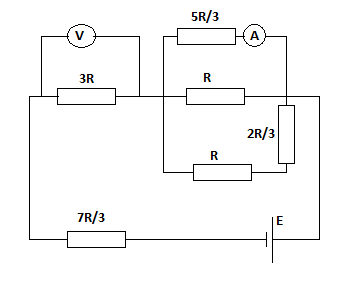For the circuit shown in the figure, both the meters are idealized, the battery has no...

Question:

For the circuit shown in the figure, both the meters are idealized, the battery has no appreciable internal resistance and the ammeter reads {eq}I {/eq}.

a. What does the voltmeter read in terms of {eq}I {/eq} and {eq}R {/eq}?

b. What is the emf E of the battery in terms of {eq}I {/eq} and {eq}R {/eq}?Currents in Parallel Circuits:

When you have branches that share two nodes, you can make use of the fact that the potential difference across the nodes is the same for all the branches. This provides a very quick way of computing the branch currents. According to Ohm's Law, the current is the ratio of the potential difference to the branch resistance.

Become a Study.com member to unlock this answer! Create your account

Part a.

The voltage {eq}V_p = \frac{5}{3}IR {/eq} in the parallel branches is the same and we can use this fact to determine the currents...Parallel Circuits: Calculating Currents with Ohm's Law

from

Chapter 9 / Lesson 6
2.6K

Understand Kirchhoff's law and Ohm's law for parallel circuits, and see how to find current in a parallel circuit using the parallel circuit current formula.